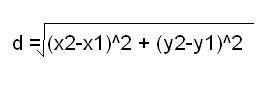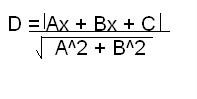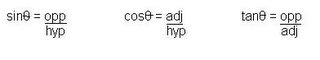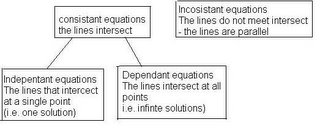<body><script type="text/javascript"> function setAttributeOnload(object, attribute, val) { if(window.addEventListener) { window.addEventListener('load', function(){ object[attribute] = val; }, false); } else { window.attachEvent('onload', function(){ object[attribute] = val; }); } } </script> <div id="navbar-iframe-container"></div> <script type="text/javascript" src="https://apis.google.com/js/platform.js"></script> <script type="text/javascript"> gapi.load("gapi.iframes:gapi.iframes.style.bubble", function() { if (gapi.iframes && gapi.iframes.getContext) { gapi.iframes.getContext().openChild({ url: 'https://draft.blogger.com/navbar.g?targetBlogID\x3d14084555\x26blogName\x3dPre-Cal+30S\x26publishMode\x3dPUBLISH_MODE_BLOGSPOT\x26navbarType\x3dBLUE\x26layoutType\x3dCLASSIC\x26searchRoot\x3dhttps://pc30s.blogspot.com/search\x26blogLocale\x3den_US\x26v\x3d2\x26homepageUrl\x3dhttps://pc30s.blogspot.com/\x26vt\x3d-2438954136380715320', where: document.getElementById("navbar-iframe-container"), id: "navbar-iframe" }); } }); </script>

## Wednesday, November 02, 2005

### late scribe

Sorry everyone this is late but my computers bin messed up for about a week nowand I'm in Mr class room right now doing yesterday's blog.

So yesterday we did a lot of dictionary writing in the beginning of class.We started with an an Analytic Geometry toolkit.

The Pythagorean Theorem = a2 + b2 = c2

Slope of a line = rise/run =y2 - y1/x2 - x1 =y1 - y2/x1 - x2
(a.k.a rate of change)
Mid point of a line segment mid = (x1 - x2/2,y1 - y2/2)Distance between two points

Perpendicular (shortest) distance line: Ax + By + C = 0
between a line and a point Point: (x,y)Equation of Lines
-General from = Ax + By + C = 0
-Slope intercept form = y = mx + b
*point slope form = y - y1 = m(x - x1)
-if two lines are parallel
with slopes of m1 & m2 = m1 = m2
-if two lines are Perpendicular
with slopes m1 & m2 = (m1)(m2) = -1

Some trig we should knowSine Law = sinA/a = sinB/b = sinC/c

Cosine Law = a2 = b2 + c2 - 2bc cos

A System of liner equations
2 lines meet at:
*One point
*All points
*No pointsThen after all that we did a quiz and that was class.
And I'm doing todays blog to so ill say who's the next scribe then.